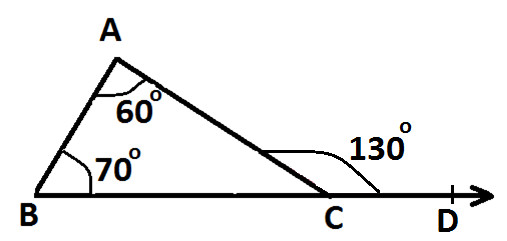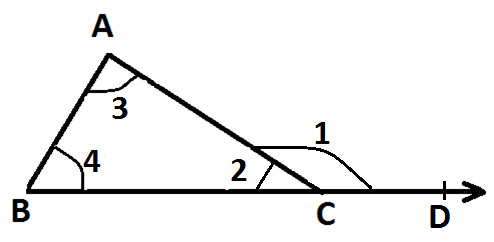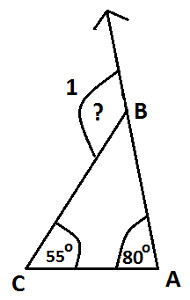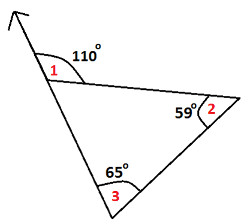Arithmetic
Arithmetic Progression
Associative Property
Averages
Brackets
Closure Property
Commutative Property
Conversion of Measurement Units
Cube Root
Decimal
Distributivity of Multiplication over Addition
Divisibility Principles
Equality
Exponents
Factors
Fractions
Fundamental Operations
H.C.F / G.C.D
Integers
L.C.M
Multiples
Multiplicative Identity
Multiplicative Inverse
Numbers
Percentages
Profit and Loss
Ratio and Proportion
Simple Interest
Square Root
Unitary Method
Algebra
Cartesian System
Order Relation
Polynomials
Probability
Standard Identities & their applications
Transpose
Geometry
Basic Geometrical Terms
Circle
Curves
Angles
Define Line, Line Segment and Rays
Non-Collinear Points
Parallelogram
Rectangle
Rhombus
Square
Three dimensional object
Trapezium
Triangle
Trigonometry
Trigonometry Ratios
Data-Handling
Arithmetic Mean
Frequency Distribution Table
Graphs
Median
Mode
Range

Videos
Solved Problems
Home >> Triangle >> Properties >> Exterior Angle Property of a Triangle >>

## Exterior Angle Property of a Triangle

 Sum of Two Sides Angle Sum Property Angles opposite to equal sides of triangle are equal Angle opposite to longer side is greater Pythagoras Theorem Exterior Angle Property of a Triangle Mid point property of Triangle Triangles on same base & between same parallel lines

Before you study exterior angle property of a triangle, you are advised to read:

What are Interior Angles of a Triangle ?
What are Exterior Angles of a Triangle
What are Interior Opposite Angles ?

Exterior Angle Property of a Triangle states that measure of exterior angle of a triangle is equals to the sum of measures of its interior opposite angles.

In order words:

Exterior Angle = Sum of Interior Opposite Angles

As shown in the following diagram:Exterior Angle is ∠ ACD and its two interior opposite angles are ∠ BAC and ∠ ABC
Measure of ∠ ACD = 130°
Measure of one interior ∠ BAC = 60°
Measure of other interior ∠ ABC = 70°

Now, we can observe that:
130° = 60° + 70°
Or we can say:
∠ ACD = ∠ BAC and ∠ ABC

Hence, its demonstrated that measure of exterior angle of a triangle is equals to the sum of measures of its interior opposite angles

Now let's prove/justify External Angle Property of a Triangle:

Before we prove/justify this topic, you are advised to read:

What is Angle Sum Property of a Triangle ?
What is Linear Pair ?

External Angle Property of a Triangle is proved or justified as follows:

In the following diagram:∠ 1 = External Angle
∠ 2, ∠ 3 & ∠ 4 = Interior Angles or we can also refer them as Angles of Triangle ABC
∠ 3 & ∠ 4 = Interior Opposite Angles to Exterior ∠ 1

Now, as per Angle Sum Property:
∠ 2 + ∠ 3 + ∠ 4 = 180°
Or
∠ 2 = 180° - ∠ 3 - ∠ 4 ..... (Statement 1)

And, we can see that ∠ 1 & ∠ 2 form a Linear Pair, therefore:
∠ 1 + ∠ 2 = 180°
Or
∠ 1 = 180° - ∠ 2

Put values of ∠ 2 from statement 1 and we get:
∠ 1 = 180° - (180 - ∠ 3 - ∠ 4)

Open brackets and we get:
∠ 1 = 180 - 180 + ∠ 3 + ∠ 4

Solve R.H.S and we get
∠ 1 = ∠ 3 + ∠ 4 ..... (statement 2)

Now as mentioned above:
∠ 1 = External Angle
∠ 3 & ∠ 4 = Interior Opposite Angles to Exterior ∠ 1
Put these into statement 2 and we get:
Exterior ∠ 1 = Interior Opposite ∠ 3 + Interior Opposite ∠ 4

Hence, proved:
Exterior = Sum of Interior Opposite Angles

From this property we can also conclude that:
Exterior Angle of a Triangle is Greater Than either of its Interior Opposite Angles.

### Study More Solved Questions / Examples

 In the following Triangle ABC, find measure of exterior ∠ 1:Find measure of exterior angle whose interior opposite angles are 50° & 75° Find error in the following diagram:Copyright@2022 Algebraden.com (Math, Algebra & Geometry tutorials for school and home education)